# Elliptic hyperboloid of one sheet

## Definition

The surface type is not unique up to isometry or even up to similarity transformations, but rather, depends on multiple nonzero parameters$a,b,c$. If we're considering the surface up to rigid isometries, the parameters are unique up to transposition of$a$ and$b$, which we can avoid by stipulating that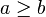$a \ge b$.

The surface, however, is unique up to affine transformations, which include transformations that do not preserve the affine structure.

### Implicit and parametric descriptions

Degree of generality Implicit description What the parameters mean Parametric description What the additional parameters mean Comment
Arbitrary Fill this in later This version need not be centered at the origin and need not be oriented parallel to the axes.
Up to rotations$\frac{(x - x_0)^2}{a^2} + \frac{(y - y_0)^2}{b^2} - \frac{(z - z_0)^2}{c^2} = 1$$a,b,c$ are positive numbers representing the semi-axis lengths.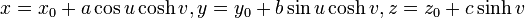$x = x_0 + a\cos u \cosh v, y = y_0 + b \sin u \cosh v, z = z_0 + c \sinh v$ This version need not be centered at the origin but is oriented parallel to the axes.
Up to rigid motions (rotations, translations, reflections)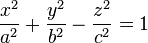$\frac{x^2}{a^2} + \frac{y^2}{b^2} - \frac{z^2}{c^2} = 1$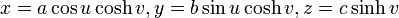$x = a\cos u \cosh v, y = b \sin u \cosh v, z = c \sinh v$
Up to similarity transformations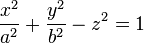$\frac{x^2}{a^2} + \frac{y^2}{b^2} - z^2 = 1$ We ca normalize$c$ to 1 using a similarity transformation.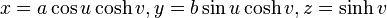$x = a\cos u \cosh v, y = b \sin u \cosh v, z = \sinh v$
Up to all affine transformations (not permissible if we want to study geometric structure)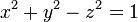$x^2 + y^2 - z^2 = 1$

## Basic topology

Topologically, the elliptic hyperboloid of one sheet is homeomorphic to the infinite right circular cylider. It is a non-compact regular surface. it divides its complement in$\R^3$ into two pieces, one of which is homeomorphic to$\R^3$ and the other is homeomorphic to the complement of a line in$\R^3$.

## Ruling

The elliptic hyperboloid of one sheet is a ruled surface, i.e., every point on the surface is contained in a line that also lies on the surface.

Below is an explicit parametrization using a ruling:

Fill this in later

## Particular cases

In the case$a = b$, we get a circular hyperboloid of one sheet.